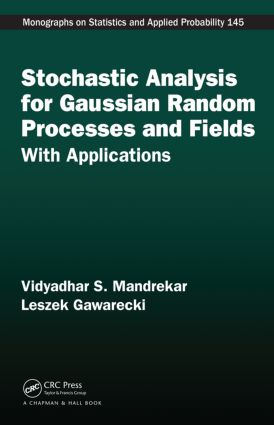Stochastic Analysis for Gaussian Random Processes and Fields

With Applications, 1st Edition

Chapman and Hall/CRC

201 pages

Hardback: 9781498707817
pub: 2015-06-23
\$110.00
x
eBook (VitalSource) : 9780429172311
pub: 2015-06-23
from \$28.98

FREE Standard Shipping!

Description

Stochastic Analysis for Gaussian Random Processes and Fields: With Applications presents Hilbert space methods to study deep analytic properties connecting probabilistic notions. In particular, it studies Gaussian random fields using reproducing kernel Hilbert spaces (RKHSs).

The book begins with preliminary results on covariance and associated RKHS before introducing the Gaussian process and Gaussian random fields. The authors use chaos expansion to define the Skorokhod integral, which generalizes the Itô integral. They show how the Skorokhod integral is a dual operator of Skorokhod differentiation and the divergence operator of Malliavin. The authors also present Gaussian processes indexed by real numbers and obtain a Kallianpur–Striebel Bayes' formula for the filtering problem. After discussing the problem of equivalence and singularity of Gaussian random fields (including a generalization of the Girsanov theorem), the book concludes with the Markov property of Gaussian random fields indexed by measures and generalized Gaussian random fields indexed by Schwartz space. The Markov property for generalized random fields is connected to the Markov process generated by a Dirichlet form.

Covariances and Associated Reproducing Kernel Hilbert Spaces

Covariances and Negative Definite Functions

Reproducing Kernel Hilbert Space

Gaussian Random Fields

Gaussian Random Variable

Gaussian Spaces

Stochastic Integral Representation

Chaos Expansion

Stochastic Integration for Gaussian Random Fields

Multiple Stochastic Integrals

Skorokhod Integral

Skorokhod Differentiation

Ogawa Integral

Appendix

Skorokhod and Malliavin Derivatives for Gaussian Random Fields

Malliavin Derivative

Duality of the Skorokhod Integral and Derivative

Duration in Stochastic Setting

Special Structure of Covariance and Ito Formula

Filtering with General Gaussian Noise

Bayes Formula

Zakai Equation

Kalman Filtering for Fractional Brownian Motion Noise

Equivalence and Singularity

General Problem

Equivalence and Singularity of Measures Generated by Gaussian Processes

Conditions for Equivalence: Special Cases

Prediction or Kriging

Absolute Continuity of Gaussian Measures under Translations

Markov Property of Gaussian Fields

Linear Functionals on the Space of Radon Signed Measures

Analytic Conditions for Markov Property of a Measure-Indexed Gaussian Random Field

Markov Property of Measure-Indexed Gaussian Random Fields Associated with Dirichlet Forms

Appendix A: Dirichlet Forms, Capacity, and Quasi-Continuity

Appendix B: Balayage Measure

Appendix C: Example

Markov Property of Gaussian Fields and Dirichlet Forms

Markov Property for Ordinary Gaussian Random Fields

Gaussian Markov Fields and Dirichlet Forms

Bibliography

Index

Vidyadhar Mandrekar is a professor in the Department of Statistics and Probability at Michigan State University. He earned a PhD in statistics from Michigan State University. His research interests include stochastic partial differential equations, stationary and Markov fields, stochastic stability, and signal analysis.

Leszek Gawarecki is head of the Department of Mathematics at Kettering University. He earned a PhD in statistics from Michigan State University. His research interests include stochastic analysis and stochastic ordinary and partial differential equations.